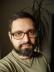## Compute the Equal Point in the Fahrenheit and Celsius Scales

I used a simple numerical method to find the equal point: Start randomly, move in one direction, if the difference is greater, change the direction, otherwise decrease the step, until there’s no difference.

Interestingly, no epsilon is needed to compare the floats, in the end they’ll be equal.

``````#!/usr/bin/perl
use warnings;
use strict;
use feature qw{ say };

sub f_to_c {
my (\$f) = @_;
(\$f - 32) / (212 - 32) * 100
}

use Test::More;
is f_to_c(32), 0;
is f_to_c(212), 100;
done_testing(2);

my \$f = rand(800) - 400;
my \$step = 100;
my \$dir = 1;
while (\$dir) {
my \$c = f_to_c(\$f);
\$f += \$dir * \$step;
\$step /= 2 if \$dir == (\$f <=> \$c);
\$dir = (\$c <=> \$f);
}
printf "%.4f\n", \$f;``````

The result is -40.0000.

## Identity Matrix

When you hear “matrix in Perl”, think PDL. It can handle large multidimensional data, so matrices are just a small part of its domain, but this week’s challenge shows clearly how easily you can solve problems in PDL.

``````#!/usr/bin/perl
use warnings;
use strict;

use PDL;
use PDL::MatrixOps;

print identity(shift);``````

If you don’t work with PDL regularly, you might notice that the most problematic task is to find the appropriate function in the documentation.I blog about Perl.